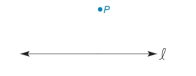Chapter 2.3, Problem 35E### Elementary Geometry for College St...

6th Edition
Daniel C. Alexander + 1 other
ISBN: 9781285195698

#### Solutions

Chapter
Section### Elementary Geometry for College St...

6th Edition
Daniel C. Alexander + 1 other
ISBN: 9781285195698
Textbook Problem
1 views

# Given that point P does not lie on the line l , construct the line through point P that is parallel to line l .To determine

To Construct:

The parallel line.

Explanation

Given:

A line 𝓁 and a point P that does not lie on 𝓁.

Figure (1)

If two lines are cut by a transversal so that a pair of corresponding angles is congruent, then these lines are parallel.

Construction:

Step (1): Consider an arbitrary point Q on the line 𝓁.

Figure (2)

Step (2): Draw a line passing through point P and point Q to become transversal

### Still sussing out bartleby?

Check out a sample textbook solution.

See a sample solution

#### The Solution to Your Study Problems

Bartleby provides explanations to thousands of textbook problems written by our experts, many with advanced degrees!

Get Started

#### The radius of convergence of is: 1 3 ∞

Study Guide for Stewart's Single Variable Calculus: Early Transcendentals, 8th

#### True or False: If F is conservative, then curl F = 0.

Study Guide for Stewart's Multivariable Calculus, 8th

#### Graph each equation. 3x5y=3(x5)

College Algebra (MindTap Course List)